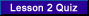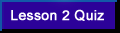VA FileMan V. 22 Key and Index Tutorial[<-- Previous Lesson][Next Lesson -->]

Part 1 - Regular Indexes

 Lesson 2. Print the Definition of Your New Index to View Data

VA FileMan provides several data dictionary listing formats to view the definition of a file. In Version 22, the Standard data dictionary listing has been modified to display New-style indexes. In addition, a new Indexes Only type of listing displays only the cross-references, both new and Traditional, as they are defined on a file.

Exercise 2.1.  See the New-Style Index You Created in Lesson 1

 Step 1. First, follow the menu path to the data dictionary listing option List File Attributes:
 VA FileMan   Data Dictionary      List File Attributes

 Step 2. Select your ZZINDIVIDUAL test file:
 ```START WITH WHAT FILE: ZZINDIVIDUAL GO TO WHAT FILE: ZZINDIVIDUAL// Select SUB-FILE: Select LISTING FORMAT: STANDARD// ?? Choose from: 1 STANDARD 2 BRIEF 3 CUSTOM-TAILORED 4 MODIFIED STANDARD 5 TEMPLATES ONLY 6 GLOBAL MAP 7 CONDENSED 8 INDEXES ONLY 9 KEYS ONLY```Select LISTING FORMAT: STANDARD// INDEXES ONLY

 Step 3. At the "Device:" prompt, enter ;80;999 to display the report on your terminal:
 ``` DEVICE: ;80;999 The following is a report of all the cross-references defined on the ZZINDIVIDUAL test file: INDEX AND CROSS-REFERENCE LIST -- FILE #662nnn 09/07/00 PAGE 1 ------------------------------------------------------------------ File #662nnn Traditional Cross-References: B REGULAR Field: NAME (662nnn,.01) 1)= S ^DIZ(662nnn,"B",\$E(X,1,30),DA)="" 2)= K ^DIZ(662nnn,"B",\$E(X,1,30),DA) New-Style Indexes: C (#nnn) RECORD REGULAR IR LOOKUP & SORTING Short Descr: This is a Regular index on the DOB and SSN fields. Set Logic: S ^DIZ(662nnn,"C",X(1),\$E(X(2),1,30),DA)="" Kill Logic: K ^DIZ(662nnn,"C",X(1),\$E(X(2),1,30),DA) Whole Kill: K ^DIZ(662nnn,"C") X(1): DOB (662nnn,.03) (Subscr 1) (forwards) X(2): SSN (662nnn,.02) (Subscr 2) (Len 30) (forwards) Subfile #662nnn.02 Traditional Cross-References: B REGULAR Field: ALIAS (662nnn.02,.01) 1)= S ^DIZ(662nnn,DA(1),2,"B",\$E(X,1,30),DA)="" 2)= K ^DIZ(662nnn,DA(1),2,"B",\$E(X,1,30),DA)```

How to Look at the Data in Your Index

From programmer mode, do a global listing and look at the data in the "C" index of the ZZINDIVIDUAL file as shown below:

 ```>D ^%G Global ^DIZ(662nnn,"C" DIZ(662nnn,"C" ^DIZ(662nnn,"C",2191031,"012345678",9) = ^DIZ(662nnn,"C",2320205,555443333,2) = ^DIZ(662nnn,"C",2380409,765432123,10) = ^DIZ(662nnn,"C",2450520,234567890,13) = ^DIZ(662nnn,"C",2450520,333221111,1) = ^DIZ(662nnn,"C",2470522,444996666,12) = ^DIZ(662nnn,"C",2480811,345678901,7) = ^DIZ(662nnn,"C",2550602,777889999,6) = ^DIZ(662nnn,"C",2590714,888776666,3) = ^DIZ(662nnn,"C",2691123,111223333,4) = ^DIZ(662nnn,"C",2700321,678765432,11) = ^DIZ(662nnn,"C",2710227,987654321,5) = ^DIZ(662nnn,"C",2730926,543891234,8) = Global ^```
 As you can see, the first subscript after the index name "C" contains the data from the DOB field. The next subscript contains the data from the SSN field. The last subscript in the index is the internal entry number of the indexed record.Prior to Version 22, the only way to create an index such as this was to create two MUMPS cross-references, one on the DOB field, and one on the SSN field. In fact, New-style Regular indexes are so powerful, the only time you should need to create a MUMPS cross-reference is for a cross-reference that performs some action, rather than sets an index.

End of Exercise 2.1.Select this link to test yourself on what you've learned in this lesson.

 [<-- Previous Lesson]   [Intro]  2            [Next Lesson -->]

Reviewed/Updated: March 20, 2007